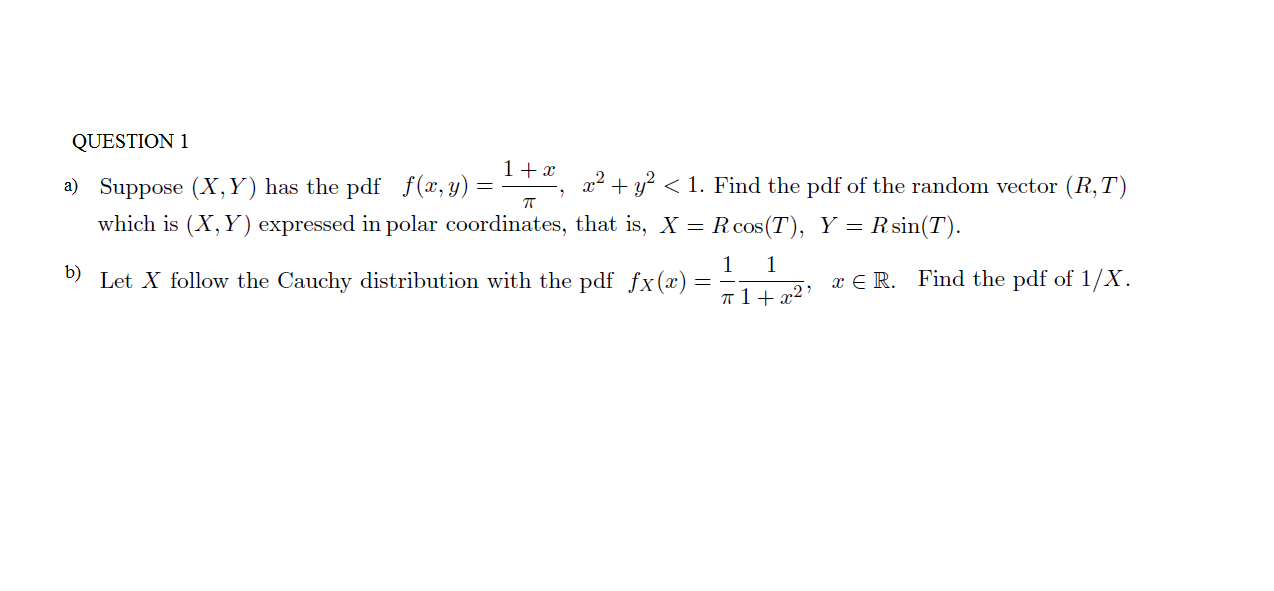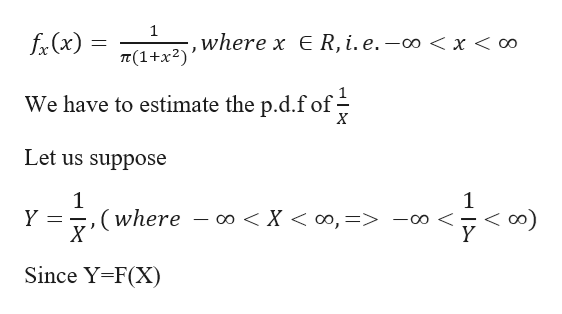# QUESTION 11 +xx1. Find the pdf of the random vector (R,Ta) Suppose (X, Y) has the pdf f (x, y) =Rcos (T) Y Rsin(T)which is (X, Y) expressed in polar coordinates, that is, X =1Let X follow the Cauchy distribution with the pdf fx(x)1b)Find the pdf of 1/XE R.12

Question
54 views

question (b) make it very detailedhelp_outlineImage TranscriptioncloseQUESTION 1 1 +x x 1. Find the pdf of the random vector (R,T a) Suppose (X, Y) has the pdf f (x, y) = Rcos (T) Y Rsin(T) which is (X, Y) expressed in polar coordinates, that is, X = 1 Let X follow the Cauchy distribution with the pdf fx(x) 1 b) Find the pdf of 1/X E R. 12 fullscreen
check_circle

Step 1

As per the question

Given p.d.f o...help_outlineImage Transcriptionclose1 f(x) where x € R, i. e. — 0o < x < о T(1+x2) We have to estimate the p.d.f of Let us suppose 1 (where X < oo, => -oo < Y Y oo _ X ( Since Y-F(X) V fullscreen

### Want to see the full answer?

See Solution

#### Want to see this answer and more?

Solutions are written by subject experts who are available 24/7. Questions are typically answered within 1 hour.*

See Solution
*Response times may vary by subject and question.
Tagged in

### Other